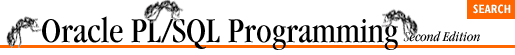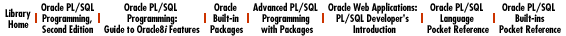home | O'Reilly's CD bookshelfs | FreeBSD | Linux | Cisco | Cisco Exam

### 7.3 The Numeric FOR Loop

There are two kinds of PL/SQL FOR loops: the numeric FOR loop and the cursor FOR loop. The numeric FOR loop is the traditional and familiar "counted" loop. The number of iterations of the FOR loop is known when the loop starts; it is specified in the loop boundary's range scheme. The number of times the loop executes is actually determined by the range scheme found between the FOR and LOOP keywords in the boundary.

The range scheme implicitly declares the loop index (if it has not already been declared), specifies the start and end points of the range, and optionally dictates the order in which the loop index proceeds (from lowest to highest or highest to lowest).

Here is the general syntax of the numeric FOR loop:

```FOR <loop index> IN [REVERSE] <lowest number> .. <highest number>
LOOP
<executable statement(s)>
END LOOP;```

You must have at least one executable statement between the LOOP and END LOOP key words.

The following table summarizes the properties of the numeric FOR loop:

Property

Description

How the loop is terminated

The numeric FOR loop terminates unconditionally when the number of times specified in its range scheme has been satisfied. You can also terminate the loop with an EXIT statement, but this is not recommended.

When the test for termination takes place

After each execution of the loop body, PL/SQL checks the value of the loop index. When it exceeds the difference between the upper and lower bounds of the range scheme, the loop terminates. If the lower bound is greater than the upper bound of the range scheme, the loop never executes its body.

Reason to use this loop

Use the numeric FOR loop when you want to execute a body of code a fixed number of times, and you do not want to halt that looping prematurely.

### 7.3.1 Rules for Numeric FOR Loops

• Do not declare the loop index. PL/SQL automatically and implicitly declares it as a local variable with datatype INTEGER. The scope of this index is the loop itself; you cannot reference the loop index outside the loop.

• Expressions used in the range scheme (both for lowest and highest bounds) are evaluated once, when the loop starts. The range is not re-evaluated during the execution of the loop. If you make changes within the loop to the variables which you used to determine the FOR loop range, those changes will have no effect.

• Never change the values of either the loop index or the range boundary from within the loop. This is an extremely bad programming practice. In most cases, PL/SQL will not let you compile such code.

• Do not use an EXIT statement inside a FOR loop in order to cause early execution of the loop. If you are going to use a numeric FOR loop, then you should let the loop execute as it is designed: from start value to end value. If you need more control over how frequently a loop is to execute and, particularly, when it is to terminate, do not use the FOR loop. Instead, use the WHILE loop or simple loop with EXIT WHEN constructs.

• Use the REVERSE keyword to force the loop to decrement from the upper bound to the lower bound. You must still make sure that the first value in the range specification (the N in N .. M) is less than the second value. Do not reverse the order in which you specify these values when you use the REVERSE keyword.

### 7.3.2 Examples of Numeric FOR Loops

The following examples demonstrate some variations of the numeric FOR loop syntax:

• The loop executes ten times; loop_counter starts at 1 and ends at 10:

```FOR loop_counter IN 1 .. 10
LOOP
... executable statements ...
END LOOP;```
• The loop executes ten times; loop_counter starts at 10 and ends at 1:

```FOR loop_counter IN REVERSE 1 .. 10
LOOP
... executable statements ...
END LOOP;```
• Here is a loop that doesn't execute even once. I specified REVERSE so the loop index, loop_counter, will start at the highest value and end with the lowest. I then mistakenly concluded that I should switch the order in which I list the highest and lowest bounds:

```FOR loop_counter IN REVERSE 10 .. 1
LOOP
/* This loop body will never execute even once! */
END LOOP;```

Even when you specify a REVERSE direction, you must still list the lowest bound before the highest bound. If the first number is greater than the second number, the body of the loop will not execute at all. If the lowest and highest bounds have the same value, then the loop will execute just once.

• Loop executes for a range determined by the values in the variable and expression:

```FOR calc_index IN start_period_number ..
LEAST (end_period_number, current_period)
LOOP
... executable statements ...
END LOOP;```

Note that in this example we do not know the values for the lowest and highest bounds at the time of the writing of the code. The actual or dynamic range is determined at runtime and is fully supported by PL/SQL. In other words, the numeric FOR loop needs to know when it starts and how many times it will execute, but you do not have to know this when you write the program.

### 7.3.3 Handling Nontrivial Increments

PL/SQL does not provide a "step" syntax, whereby you can specify that the loop index increment. In all variations of the PL/SQL numeric FOR loop, the loop index is always incremented or decremented by one.

If you have a loop body which you want executed for a nontrivial (different from one) increment, you will have to write some cute code. For example, what if you want your loop to execute only for all even numbers between 1 and 100? You can make use of the numeric MOD function, as follows:

```FOR loop_index IN 1 .. 100
LOOP
IF MOD (loop_index, 2) = 0
THEN
/* We have an even number, so perform calculation */
calc_values (loop_index);
END IF;
END LOOP;```

Or you can use simple multiplication inside a loop with half the iterations:

```FOR even_number IN 1 .. 50
LOOP
calc_values (even_number*2);
END LOOP;```

In both cases, the calc_values procedure executes only for even numbers. In the first example, the FOR loop executes 100 times. In the second example, the FOR loop executes only 50 times.

Whichever approach you decide to take, be sure to document this kind of technique clearly. You are, in essence, manipulating the numeric FOR loop to do something for which it is not designed. Comments would be very helpful for the maintenance programmer who has to understand why you would code something like that.7.2 The Simple Loop7.4 The Cursor FOR Loop